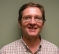## CMSIS DSP ARM Complex FFT output arrangementStarted by 4 years ago10 replieslatest reply 4 years ago1455 views

I'm trying a snippet of code, based on CMSIS DSP Library, that take 2 quadrature (I & Q) signals and do complex fft.

(pseudo code)

FFT_SIZE = 128

I signal 

Q signal 

inputBuf

S = &arm_cfft_sR_f32_len128;

arm_cfft_f32(S, inputBuf, 0, 1);

arm_cmplx_mag_f32(inputBuf, fftOut, FFT_SIZE);

As shown in several examples, I arrange the input buffer in an interleaved way [ I0, Q0, I1 , Q1 ...], treating the I signal as the "real" part , and the Q signal as the "imaginary" part.

But I'm a bit confused with the output I get.

First: what is the meaning of the Bit Reversal Flag and what is the effect of setting 0 or 1?

Second: what is the arrangement of the frequency bins in the output buffer? (where are located the DC bin, the negative frequency bins, and the positive frequency bins?)

[ - ]Bit reversal means that the output is in bit reversed order.  Bin 0 is at offset 0, Bin 1 is at offset 0x40, bin 2 is at offset 0x20, bin 3 is at offset 0x60, etc.

One way to understand is just with a series of complex tones.  For a 128 point FFT there are 128 unique frequency bins around the unit circle.  Step through all 128 and see which bin gets the energy for each frequency.

In "natural" non-bit reversed order, the 1st positive frequency should be in bin 1.  The "1st" negative frequency should be in bin N-1 (63).  The Nyquist frequency will be in bin N/2 (32).

Another thing that always trips me up for FFT/IFFT is the scale factor.  The definition is different for the two cases and is different for fixed point where there can be an optional shift at the radix stages to prevent overflow.  One way to look at it is power.  There should be the same amount of power in the frequency domain as in the time domain.

FWIW,

Mark Napier

[ - ]bit reverse order is that for 000,001,010,011 bins as example it outputs bins 000,100,010,110 i.e. reverse index bit order left/right.

This is to do with fft butterfly. It may either accept serial input order but outputs in bit reverse order or vice versa. If output is bit reverse you may want it put right at extra cost.

[ - ]I'm still a little confused with your statement: In "natural" non-bit reversed order, the 1st positive frequency should be in bin 1.  The "1st" negative frequency should be in bin N-1 (63).  The Nyquist frequency will be in bin N/2 (32).

Is therea mistake when you write N-1 (63) instead of (127), being FFT of 128 pt?

I found the following pic:Is there what I get when I set Bit Reversal to TRUE?

Thanks.

[ - ]Yes, my mistake.  N = 128.  N-1 => 127, this "1st" negative frequency.  Your picture is correct.

Note that both of your pictures are in natural order, not bit reversed.

Note that the "1st" frequency is a single sine wave period over 128 samples.  The 2nd bin holds 2 cycles, etc.

N/2 = 64 or the bin for the Nyquist frequency.

[ - ]> The definition is different for the two cases and is different ...

I would say "different for every different code library out there".  But maybe things have settled.

[ - ]may be I'm missing something here.  may i ask you one question : why are you feeding I & Q signal as Real and Imag. typical case, I & Q itself will have it's own real and imaginary.

I & Q are orthogonal complex signals think of Hilbert pairs. Re and Im signals are real signals. for example, R/Im are coming out of the complex mixer as two real output array. a comlex mixer will have two complex outputs I and Q.

hope it helps.

-Chalil

[ - ]Yes Chalil you are missing all the fundamental definitions. I and Q in communications is meant to be Re/Im. Moreover what has mixer got to do here in such definitions. a mixer can have re/im in and re/im out or call it I/Q in and out.

[ - ]In this case the application is with a Doppler Radar, where I is IF output from Mixer 1 and Q is IF output from Mixer 2 (which is fed whit a 90° delayed oscillator).

EDIT to above post: Yes, the output of cfft_f32 , with Bit Reversal == TRUE is as depicted in the image above. Then, for me, it was useful a reordering of the bins with negative frequencies on the left (IV quadrant) and positives on the right (I quadrant).
[ - ]